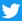## Kinematic Data:

initial
position (ft)
initial
velocity (ft/s)
average
acceleration (ft/s2)
xo = -2.251 vxo = 8.472 ax = -17.519
yo = 50.00 vyo = -137.643 ay = 35.462
zo = 5.178 vzo = -7.001 az = -13.765

Initial Speed (mph): 94.1
Speed at the front of home plate (mph): 85.3
Position at the front of home plate (ft): x = -0.314, y = 1.417, and z = 1.638

Maintained by Dr. Baseball, Ph.D. ()